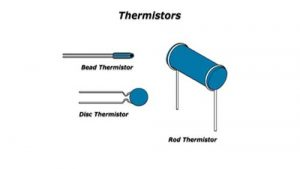# Thermistor or Time dependent resistor (TDR)

The word thermistor is a contraction of the term thermal resistor. Thermistor is also known as temperature sensitive resistor or temperature dependent resistor (TDR). It is a type of resistor whose resistance is dependent on temperature. Thermistor is used for devices, which have high temperature coefficient and used to detect very small changes in temperature. It is widely used as inrush current limiter, self resetting over current protectors, temperature sensor and self regulating heating elements. Thermistors are a type of semiconductor meaning they have greater resistance than conducting materials, but lower resistance than insulating materials. The variation in temperature is reflected through an appreciable variable of the resistance of the device. With the rise in temperature the resistance of a thermistor may increase or decrease.Thermistor can be classified depending on the temperature coefficient of resistance are,

1. ## Negative temperature coefficient (NTC) thermistor.

The negative temperature coefficient (NTC) means that the temperature increases with the decrease in resistance. It is two terminal devices. The NTC thermistors are made by sintering sintering semiconductor ceramic materials prepared from mixtures of metallic oxides of manganese, cobalt, nickel etc.

1. ## Positive temperature coefficient (PTC) thermistor.

The positive temperature coefficient (PTC) means that small change in temperature with very large change in resistance. The PTC thermistors are made by using silicon or germanium on semiconductor barium titrates.